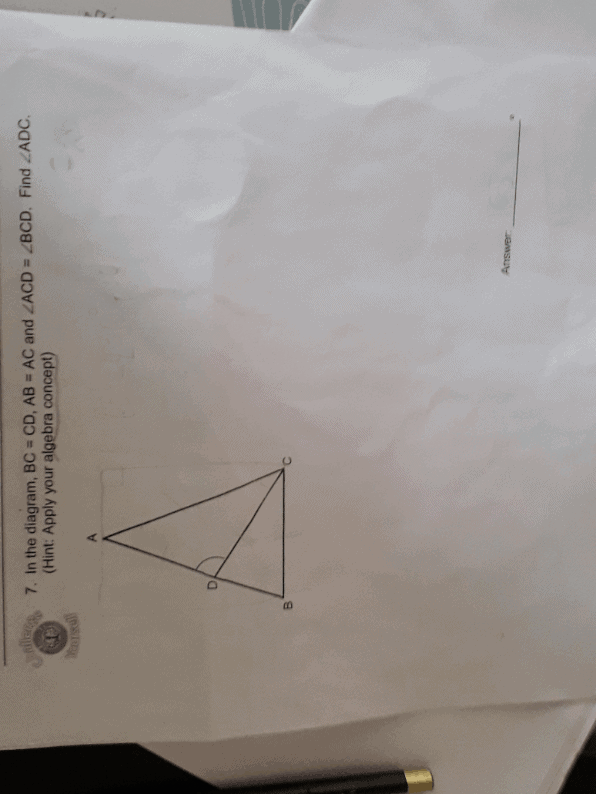# QuestionSource: ?

Let ∠ACD be y degrees.

∠BCD is also y degrees.

By virtue of isosceles triangle ABC, ∠DBC = 2 y degrees

By virtue of isosceles triangle DBC, ∠CDB = 2 y degrees

Sum of angles in triangle DBC = 5 y degrees

Hence, 5 y = 180

y = 36

∠ADC = (180 – 2 y) degrees (Sum of ∠s in a straight line ADB)

= 180 – 2 (36)

= 108 degrees//